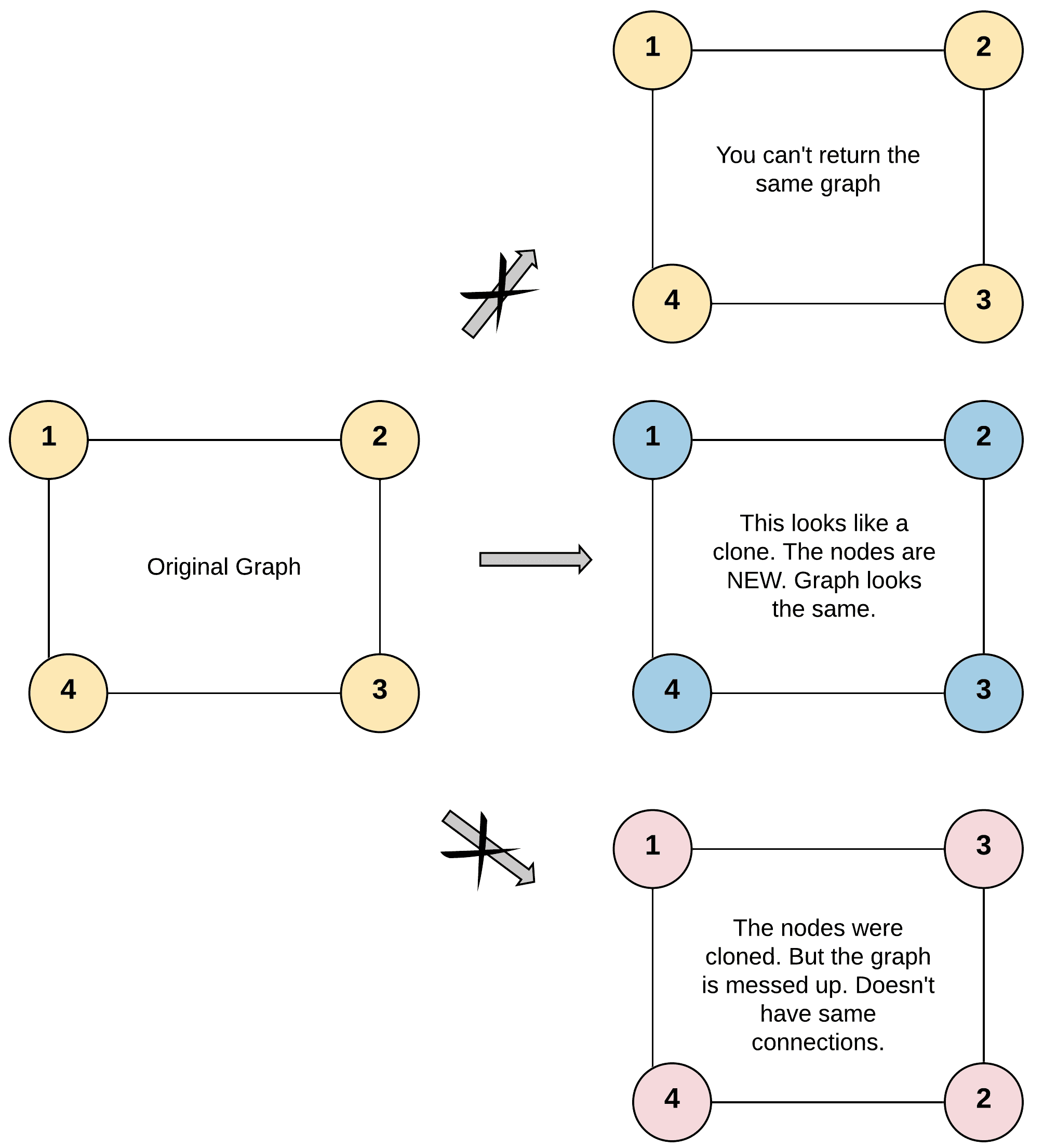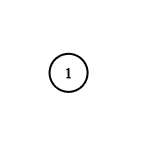# Question

Formatted question description: https://leetcode.ca/all/133.html

Given a reference of a node in a connected undirected graph.

Return a deep copy (clone) of the graph.

Each node in the graph contains a value (int) and a list (List[Node]) of its neighbors.

class Node {
public int val;
public List<Node> neighbors;
}


Test case format:

For simplicity, each node's value is the same as the node's index (1-indexed). For example, the first node with val == 1, the second node with val == 2, and so on. The graph is represented in the test case using an adjacency list.

An adjacency list is a collection of unordered lists used to represent a finite graph. Each list describes the set of neighbors of a node in the graph.

The given node will always be the first node with val = 1. You must return the copy of the given node as a reference to the cloned graph.

Example 1:Input: adjList = [[2,4],[1,3],[2,4],[1,3]]
Output: [[2,4],[1,3],[2,4],[1,3]]
Explanation: There are 4 nodes in the graph.
1st node (val = 1)'s neighbors are 2nd node (val = 2) and 4th node (val = 4).
2nd node (val = 2)'s neighbors are 1st node (val = 1) and 3rd node (val = 3).
3rd node (val = 3)'s neighbors are 2nd node (val = 2) and 4th node (val = 4).
4th node (val = 4)'s neighbors are 1st node (val = 1) and 3rd node (val = 3).


Example 2:Input: adjList = [[]]
Output: [[]]
Explanation: Note that the input contains one empty list. The graph consists of only one node with val = 1 and it does not have any neighbors.


Example 3:

Input: adjList = []
Output: []
Explanation: This an empty graph, it does not have any nodes.


Constraints:

• The number of nodes in the graph is in the range [0, 100].
• 1 <= Node.val <= 100
• Node.val is unique for each node.
• There are no repeated edges and no self-loops in the graph.
• The Graph is connected and all nodes can be visited starting from the given node.

# Algorithm

In the recursive function, it is first to check if empty, and then to see whether the current node has been cloned,

• if it exists in the HashMap, it will directly return to its mapping node.
• Otherwise, clone the current node and create a mapping in HashMap, then traverse all neihbor nodes of the current node, call the recursive function and add it to the neighbors array of the cloned node.

Same idea of HashMap for BFS solution.

# Code

• import java.util.HashMap;
import java.util.HashSet;
import java.util.Queue;
import java.util.Set;

public class Clone_Graph {

/**
* Definition for undirected graph.
* class UndirectedGraphNode {
*     int label;
*     List<UndirectedGraphNode> neighbors;
*     UndirectedGraphNode(int x) { label = x; neighbors = new ArrayList<UndirectedGraphNode>(); }
* };
*/
public class Solution {
// from original node, to its copy
HashMap<UndirectedGraphNode, UndirectedGraphNode> hm = new HashMap<>();

Set<UndirectedGraphNode> visited = new HashSet<>();

public UndirectedGraphNode cloneGraph(UndirectedGraphNode node) {

if (node == null) {
return null;
}

// it can be done in either dfs or bfs
// I prefer bfs, less space cost

q.offer(node);

while (!q.isEmpty()) {

UndirectedGraphNode currentOriginal = q.poll();
UndirectedGraphNode currentCopy = getClonedNode(currentOriginal);

for (UndirectedGraphNode nb: currentOriginal.neighbors) {

UndirectedGraphNode nbCopy = getClonedNode(nb);

/*

Input:
{0,0,0}
Output:
{0,0,0,0,0}
Expected:
{0,0,0}

*/
// @note: if add each other, then failed by above input
// 			so, should only add currentCopy's neighbors, and rest put in queue for next processing

// if (!visited.contains(nb)) {
if (!visited.contains(nb) && !q.contains(nb)) {
q.offer(nb);
}

}
}

return hm.get(node);
}

private UndirectedGraphNode getClonedNode(UndirectedGraphNode original) {

UndirectedGraphNode originalCopy;

if (!hm.containsKey(original)) {

originalCopy = new UndirectedGraphNode(original.label);
hm.put(original, originalCopy); // as long as no mapping from original to its copy, add it
} else {
originalCopy = hm.get(original);
}

return originalCopy;
}
}
}

############

/*
// Definition for a Node.
class Node {
public int val;
public List<Node> neighbors;
public Node() {
val = 0;
neighbors = new ArrayList<Node>();
}
public Node(int _val) {
val = _val;
neighbors = new ArrayList<Node>();
}
public Node(int _val, ArrayList<Node> _neighbors) {
val = _val;
neighbors = _neighbors;
}
}
*/

class Solution {
private Map<Node, Node> visited = new HashMap<>();

public Node cloneGraph(Node node) {
if (node == null) {
return null;
}
if (visited.containsKey(node)) {
return visited.get(node);
}
Node clone = new Node(node.val);
visited.put(node, clone);
for (Node e : node.neighbors) {
}
return clone;
}
}

• // OJ: https://leetcode.com/problems/clone-graph/
// Time: O(N)
// Space: O(N)
class Solution {
unordered_map<Node*, Node*> m;
public:
Node* cloneGraph(Node* node) {
if (!node) return nullptr;
if (m.count(node)) return m[node];
auto cpy = new Node(node->val);
m[node] = cpy;
for (auto &n : node->neighbors) cpy->neighbors.push_back(cloneGraph(n));
return cpy;
}
};

• """
# Definition for a Node.
class Node:
def __init__(self, val = 0, neighbors = None):
self.val = val
self.neighbors = neighbors if neighbors is not None else []
"""

class Solution:
def cloneGraph(self, node: 'Node') -> 'Node':
visited = defaultdict()

def clone(node):
if node is None:
return None
if node in visited:
return visited[node]
c = Node(node.val)
visited[node] = c
for e in node.neighbors:
c.neighbors.append(clone(e))
return c

return clone(node)

############

# Definition for a undirected graph node
# class UndirectedGraphNode:
#     def __init__(self, x):
#         self.label = x
#         self.neighbors = []

class Solution:
# @param node, a undirected graph node
# @return a undirected graph node
def cloneGraph(self, node):
graph = {}
visited = set()

def dfs(node, visited, graph):
if not node or node.label in visited:
return
visited |= {node.label}
if node.label not in graph:
graph[node.label] = UndirectedGraphNode(node.label)
newNode = graph[node.label]

for nbr in node.neighbors:
if nbr.label not in graph:
graph[nbr.label] = UndirectedGraphNode(nbr.label)
newNode.neighbors.append(graph[nbr.label])
dfs(nbr, visited, graph)
return newNode

return dfs(node, visited, graph)


• /**
* Definition for a Node.
* type Node struct {
*     Val int
*     Neighbors []*Node
* }
*/

func cloneGraph(node *Node) *Node {
visited := map[*Node]*Node{}
var clone func(node *Node) *Node
clone = func(node *Node) *Node {
if node == nil {
return nil
}
if _, ok := visited[node]; ok {
return visited[node]
}
c := &Node{node.Val, []*Node{} }
visited[node] = c
for _, e := range node.Neighbors {
c.Neighbors = append(c.Neighbors, clone(e))
}
return c
}

return clone(node)
}

• /**
* Definition for Node.
* class Node {
*     val: number
*     neighbors: Node[]
*     constructor(val?: number, neighbors?: Node[]) {
*         this.val = (val===undefined ? 0 : val)
*         this.neighbors = (neighbors===undefined ? [] : neighbors)
*     }
* }
*/

function cloneGraph(node: Node | null): Node | null {
if (node == null) return null;

const visited = new Map();
visited.set(node, new Node(node.val));
const queue = [node];
while (queue.length) {
const cur = queue.shift();
for (let neighbor of cur.neighbors || []) {
if (!visited.has(neighbor)) {
queue.push(neighbor);
const newNeighbor = new Node(neighbor.val, []);
visited.set(neighbor, newNeighbor);
}
const newNode = visited.get(cur);
newNode.neighbors.push(visited.get(neighbor));
}
}
return visited.get(node);
}


• using System.Collections.Generic;

public class Solution {
public Node CloneGraph(Node node) {
if (node == null) return null;
var dict = new Dictionary<int, Node>();
var queue = new Queue<Node>();
queue.Enqueue(CloneVal(node));
while (queue.Count > 0)
{
var current = queue.Dequeue();
var newNeighbors = new List<Node>(current.neighbors.Count);
foreach (var oldNeighbor in current.neighbors)
{
Node newNeighbor;
if (!dict.TryGetValue(oldNeighbor.val, out newNeighbor))
{
newNeighbor = CloneVal(oldNeighbor);
queue.Enqueue(newNeighbor);
}
}
current.neighbors = newNeighbors;
}
return dict[node.val];
}

private Node CloneVal(Node node)
{
return new Node(node.val, new List<Node>(node.neighbors));
}
}